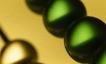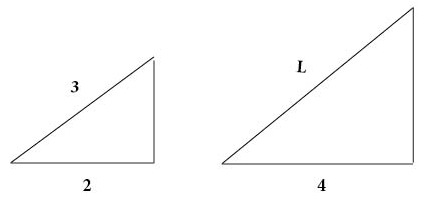## Ratio and ProportionRatio
Ratio refers to a comparison between two similar things.

Ratio can be represented with a colon.
a:b

Ratio can also be represented as a fraction.$\bf\displaystyle\frac{a}{b}$Ratio of yellow beads to green beads = 1 to 2 = 1:2 =$\bf\displaystyle\frac{1}{2}$Ratio of yellow beads to green beads = 2 to 4 = 2:4 =$\bf\displaystyle\frac{2}{4}$Ratio of yellow beads to green beads = 3 to 6 = 3:6 =$\bf\displaystyle\frac{3}{6}$

Proportion
Proportion refers to two equal ratios.
a:b = c:d$\bf\displaystyle\frac{a}{b}$$\bf\displaystyle\frac{c}{d}$

For ratios$\bf\displaystyle\frac{a}{b}$ and$\bf\displaystyle\frac{c}{d}$ to be in proportion

To Prove Ratios are in Proportion, Cross-Multiply

In order to cross-multiply, it is helpful to understand algebraic equations.
It is also helpful to know your multiplication and division tables.

For ratios$\bf\displaystyle\frac{1}{2}$ and$\bf\displaystyle\frac{6}{12}$
bc = 2 × 6
1 × 12 = 2 × 6
12 = 12
Because ad = bc, ratios$\bf\displaystyle\frac{1}{2}$ and$\bf\displaystyle\frac{6}{12}$ are in proportion.

To Solve for an Unknown Variable in Proportionate Ratios, Cross-Multiply
In order to cross-multiply, it is helpful to understand algebraic equations.
It is also helpful to know your multiplication and division tables.

For ratios$\bf\displaystyle\frac{2}{4}$ and$\bf\displaystyle\frac{5}{d}$
bc = 4 × 5
2 × d = 4 × 5
2d = 20$\bf\displaystyle\frac{2d}{2}$$\bf\displaystyle\frac{20}{2}$
d = 10

Question

Prove that the ratios of yellow to green beads in the image below are in proportion.$\bf\displaystyle\frac{1}{2}$$\bf\displaystyle\frac{2}{4}$

For ratios$\bf\displaystyle\frac{1}{2}$ and$\bf\displaystyle\frac{2}{4}$
bc = 2 × 2
1 × 4 = 2 × 2
4 = 4
Because ad = bc, ratios$\bf\displaystyle\frac{1}{2}$ and$\bf\displaystyle\frac{2}{4}$ are in proportion.

Question

In the image below, two ramps are in proportion.  Find the length L of the larger ramp.6

For ratios$\bf\displaystyle\frac{2}{3}$ and$\bf\displaystyle\frac{4}{L}$
bc = 3 × 4
2 × L = 3 × 4
2L = 12$\bf\displaystyle\frac{2L}{2}$$\bf\displaystyle\frac{12}{2}$
L = 6

Question

In the image below, two ramps are in proportion.  Find the length Q of the smaller ramp.10

For ratios$\bf\displaystyle\frac{20}{40}$ and$\bf\displaystyle\frac{5}{Q}$
bc = 40 × 5
20 × Q = 40 × 5
20Q = 200$\bf\displaystyle\frac{20Q}{20}$$\bf\displaystyle\frac{200}{20}$
Q = 10

Practice – Questions
1.  Prove that the ratios of yellow to green beads in the image below are in proportion.2.  In the image below, two ramps are in proportion.  Find the length L of the larger ramp.3.  In the image below, two ramps are in proportion.  Find the length Q of the smaller ramp.4.  In the image below, two rectangular boxes are in proportion.  Find the length L of the larger box.5.  In the image below, two rectangular boxes are in proportion.  Find the width W of the smaller box.1.  For ratios$\bf\displaystyle\frac{1}{2}$ and$\bf\displaystyle\frac{3}{6}$
Because ad = bc, ratios$\bf\displaystyle\frac{1}{2}$ and$\bf\displaystyle\frac{3}{6}$ are in proportion.